# KSEEB Solutions for Class 9 Maths Chapter 12 Circles Ex 12.6

## Karnataka Board Class 9 Maths Chapter 12 Circles Ex 12.6

Question 1.
Prove that the line of centres of two intersecting circles subtends equal angles at the two points of intersection.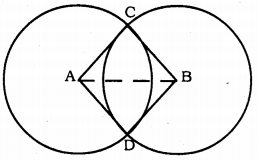Solution:
Data: Two circles having centres A and B, intersect at C and D.
To Prove: ∠ACB = ∠ADB.
Construction: Join A and B.
Proof: In ∆ABC and ∆ABD,
AC = AD (∵ radii of same circle are equal)
BC = DD
AB is common.
∴ ∆ABC ≅ ∆ABD (SSS Postulate.)
∴ ∠ACB = ∠ADB.

Question 2.
Two chords AB and CD of lengths 5 cm and 11 cm respectively of a circle are parallel to each other and are on opposite sides of its centre. If the distance between AB and CD is 6 cm., find the radius of the circle.Solution:
Two chords AB and CD of lengths 5 cm and 11 cm respectively of a circle are parallel to each other and are on opposite sides of its centre.
Distance between AB and CD is 6 cm.
To Prove: Radius of the circle, OP =?
Construction: Join OP and OQ, OB, and OD.
Proof: Chord AB || Chord CD.
AB = 5 cm, and CD =11 cm.
OP ⊥ AB
∴ BP = AP = $$\frac{5}{2}$$ = 2.5 cm.
OQ⊥CD
∴ CQ = QD = $$\frac{11}{2}$$ = 5.5 cm.
PQ = 6 cm. (Data)
Let OQ = 2 cm then, OP = (6 – x) cm.
In ∆BPO, ∠P = 90°
As per Pythagoras theorem,
OB2 = BP2 + PO2
= (2.5)2 + (6 – x)2
= 6.25 + 36 – 12x + x2
OB2 = x2– 12x + 42.25 …………….. (i)
In ∆OQD, ∠Q= 90°
∴ OD2 = OQ2 + QD2
= (x)2 + (5.5)2
OD2 = x2 + 30.25 ……………….. (ii)
OB = OD (∵ radii of same circle)
From (i) and (ii).
x2 – 12x + 42.25 = x2 + 30.25
-12x = 30.25 – 42.25
-12x = -12
12x = 12
∴ x = $$\frac{12}{12}$$
∴ x = 1 cm.
From (ii),
OD2 = x2 + 30.25
= (1)2 + 30.25
= 1 + 30.25
∴ OD2 = 31.25
OD = $$\sqrt{31.25}$$
∴ OD = 5.59 cm.
∴ Radius of circle OP = OD = 5.59 cm.

Question 3.
The lengths of two parallel chords of a circle are 6 cm and 8 cm. If the smaller chord is at distance 4 cm, from the centre, what is the distance of the other chord from the center?Solution:
Data: Chords of a circle are 6 cm. and 8 cm. are parallel. The smaller chord is at distance of 4 cm. from the centre.
To Prove: Distance between bigger chord and centre =?
Construction: Join OA and OC.
Proof: AB || CD, AB = 6 cm, CD = 8 cm.
OP⊥CD, OQ⊥CD.
In ∆OPA, ∠P = 90°
∴ OA2 = OP2 + PA2 (According to Pythagoras theorem)
= (4)2 + (3)2 = 16 + 9
OA2 = 25
∴ OA = 5 cm.
OA = OC = 5 cm. (radii of the same circle.)
Now, in ∆OQC,
OC2 = OQ2 + QC2
(5)2 = x2 + (4)2
25 = x2 + 16
x2 = 25 – 16 = 9
∴ x = $$\sqrt{9}$$ ∴ x = 3 cm.
∴ Bigger chord is at a distance of 3 cm from the centre.

Question 4.
Let the vertex of an angle ABC be located outside a circle and let the sides of the angle intersect equal chords AD and CE with the circle. Prove ∠ABC is equal to half the difference of the angles subtended by the chords AC and DE at the centre.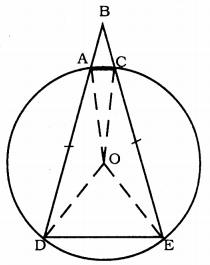Solution:
Data: The vertex of angle ABC be located outside a circle and let the sides of the angle intersect equal chords AD and CE with the circle.
To Prove: ∠ABC is equal to half the difference of the angles subtended by the chords AC and DE at the centre. OR
∠ABC= $$\frac{1}{2}$$ [∠DOE – ∠AOC].
Construction: OA, OC, OE, OD are joined.
Proof: In ∆AOD and ∆COE,
OA = OC, OD = OE radii of same circle.
AD = CE (Data)
∴ ∆AOD ≅ ∆COE (SSS Postulate)
∠OAD = ∠OCE ……….. (i)
∴∠ODA = ∠OEC …………. (ii)
OA = OD
∴ ∠OAD = ∠ODA …………. (iii)
From (i) and (ii),
∠OAD = ∠OCE = ∠ODA = ∠OEC = x°.
In ∆ODE, OD = OE
∠ODE = ∠OED = y°.
∴ ∠CAD + ∠DEC = 180°
x + a + x + y = 180
2x + a + y = 180
y = 180 – 2x – a ……….. (iv)
But, ∠DOE = 180 – 2y
∠AOC = 180 – 2aQuestion 5.
Prove that the circle drawn with any side of a rhombus as diameter passes through the point of intersection of its diagonals.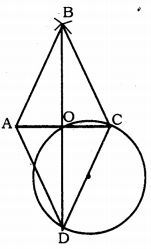Solution:
Data: ABCD is a rhombus. Circle is drawn taking side CD diameter. Let the diagonals AC and BD intersect at ‘O’.
To Prove: Circle passes through the point ‘O’ of the intersection of its diagonals.
Proof: ∠DOC = 90° (Angle in the semicircle) and diagonals of rhombus bisect at right angles at ‘O’.
∴ ∠DOC = ∠COB = ∠BOA = ∠AOD = 90°
∴ Circle passes the point of intersection of its diagonal through ‘O’.

Question 6.
ABCD is a parallelogram. The circle through A, B and C intersect CD (produced if necessary) at E. Prove that AE = AD.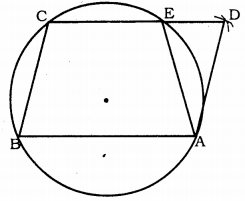Solution:
Data: ABCD is a parallelogram. The circle through A, B, and C intersect CD at E. AE is joined.
To Prove: AE = AD
Proof: ∠AEC + ∠AED = 180° …………. (i) (linear pair)
ABCE is a cyclic quadrilateral.
∴ ∠ABC + ∠AEC = 180° ………….(ii) (opposite angles)
Comparing (i) and (ii),
∠AEC = ∠AED = ∠ABC + ∠AEC
∠AED = ∠ABC ………….. (iii)
But, ∠ABC = ∠ADE (Opposite angles of quadrilateral)
Substituting in equation (iii),
∴ AE = AD.

Question 7.
AC and BD are chords of a circle which bisect each other. Prove that
(i) AC and BD are diameters,
(ii) ABCD is a rectangle.
Solution:
Data : AC and BD are chords of a circle bisect each other at ‘O’.
To Prove:
i) AC and BD are diameters.
ii) ABCD is a rectangle.
Construction: AB, BC, CD and DA are joined.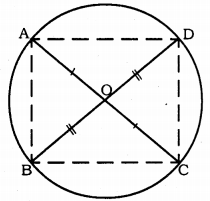Proof: In ∆AOB and ∆COD,
AO = OC (Data)
BO = OD (Data)
∠AOB = ∠COD (vertically opposite angles)
∴ ∆AOB ≅ ∆COD (SAS postulate)
∴ ∠OAB = ∠OCD
These are pair of alternate angles.
∴ AB || CD and AB = CD.
∴ ABCD is a parallelogram.
∴ ∠BAD = ∠BCD (Opposite angles of parallelogram)
But. ∠BAD + ∠BCD = 180 (∵ Angles of cyclic quadrilateral)
∴ ∠BAD = $$\frac{180}{2}$$
∴ ∠BAD = 90°.
If angles of a quadrilateral are right angles it is rectangle. ABCD is a recrtangle.
∠BAD is separated from chord BD.
∴ This is the angl in semicircle.
∴ Chord BD is a diameter.
Similarly, ∠ADC = 90°
∴ Chord AC is a diameter.

Question 8.
Bisectors of angles A, B and C of a triangle ABC intersect its circumcircle at D, E and F respectively. Prove that the angles of the triangle DEF are 90° – $$\frac{1}{2}$$ A, 90° – $$\frac{1}{2}$$ B and 90° – $$\frac{1}{2}$$ C.
Solution:
Data: AD, BE and CF are angular bisectors of angles A, B and C of ∆ABC intersects its circumference at D, E and F respectively.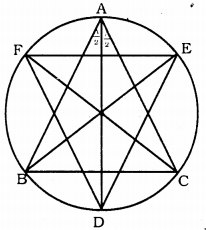To prove: Angles of ∆DEF are 90° – $$\frac{1}{2}$$ A, 90° – $$\frac{1}{2}$$ B and 90° – $$\frac{1}{2}$$ C.
Proof: AD, BE and CF are angular bisectors of angles A, B and C of ∆ABC.
∴ ∠BAD = ∠CAD = $$\frac{\angle \mathrm{A}}{2}$$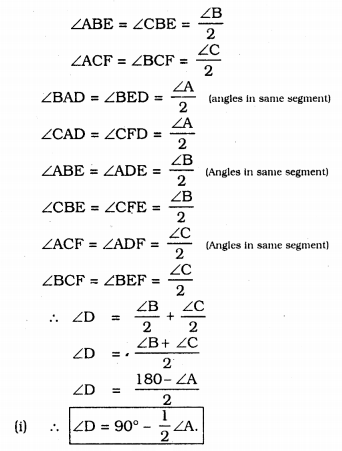Question 9.
Two congruent circles intersect each other at points A and B. Through A any line segment PAQ is drawn so that P, Q lies on the two circles.
Prove that BP = BQ.Solution:
Data : Two congruent circles intersect each other at points A and B. Through A any line segment PAQ is drawn so that P, Q lie on the two circles.
To Prove: BP = BQ
Construction: Join AB.
Proof: Two congruent triangles with centres O and O’ intersects at A and B. Through A segment PAQ is drawn so that P, Q lie on the two circles.
Similarly, ∠AQB= 70° in circle subtended by chord AB. Because Angles subtended by circumference by same chord.
∴ ∠APB = ∠AQB = 70°.
Now, in ∆PBQ, ∠QPB = ∠PQB.
∴ Sides opposite to each other are equal.
∴ BP = BQ.

Question 10.
In any triangle ABC, if the angle bisector of ∠A and perpendicular bisector of BC intersect, prove that they intersect on the circumcircle of the triangle ABC.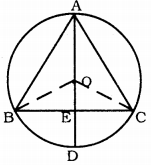Solution:
Data: In ∆ABC, if the angle bisector of ∠A and perpendicular bisector of BC intersect each other. O is the centre of the circle.
To Prove: Angle bisector of ∠A and perpendicular bisector of BC intersect at D.
Construction: Join OB, OC.
Proof: Angle subtended at the Centre
= 2 × angles subtended in the circumference.
∠BOC = 2 × ∠BAC
In ∆BOE and ∆COE,
∠OEB = ∠OEC = 90° (∵ OE⊥BC)
∴ BO = OC (radii)
OE is common.
∴ ∆BOE ≅ ∆COE (RHS postulate)
But, ∠BOE + ∠COE = ∠BOC
∠BOE + ∠BOE = ∠BOC
2∠BOE = ∠BOC
2∠BOE = 2∠BAC
∴ ∠BOE = ∠BAC
But, ∠BOE = ∠COE = ∠BAC
∠BAD = $$\frac{1}{2}$$ ∠BAC
∠BAD = $$\frac{1}{2}$$ ∠BOE
∠BAD = $$\frac{1}{2}$$ ∠BOD
∴ ∠BOD = 2∠BAD
∴ The angle subtended by an arc at the centre is double the angle subtended by it at any point on the circumference.
∴ Angle bisector of ∠A and perpendicular bisector of BC intersect at D.

##### KSEEB Solutions for Class 9 Maths

error: Content is protected !!